Overview: In Oracle HCM Cloud , Voluntary deduction is mainly a direct amount deduction specified on element entry page. There can be a scenario where an employee has deductions with rate defined. The default voluntary deduction element creation does not have option to create the rate as input value and respectively the default fast formula processing does not have the option of deduction amount calculation with rate value. This article will explain the process of voluntary deduction element with input value as Rate and calculation on the rate and amount specified on element entry page in Oracle HCM Cloud. Steps involved are listed below

Create the Voluntary Deduction Element.

Element Name: “PAY Voluntary Deduction Rate Test”Default voluntary deduction element creation does not have Rate as input value. The reason is Element Input list doesn’t have Rate.Modify the element with additional input value as Rate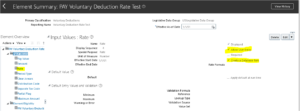This additional input needs to process through fast formula. Hence fast formula specified on “Status Processing Rules” should be modified to consider the additional input value.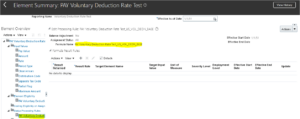Add the input value as Rate in fast formula to push the rate value to the array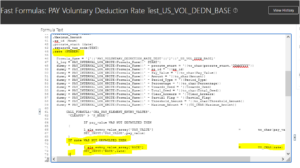The shadow element Calculator uses the different fast formula to calculate the input value and return the value to Result element.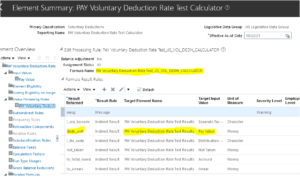This fast formula should be modified to get the rate value to do the deduction calculation.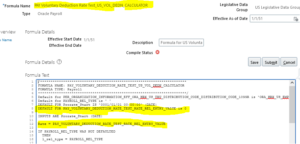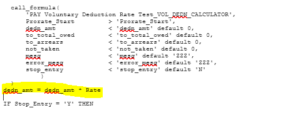The changes at element have been completed.

Verify the payroll run result with Element Entry page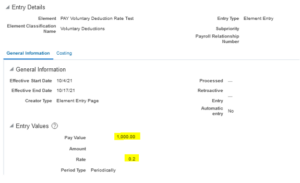Quick Pay result of this element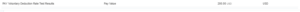Result == 1000 * 0.2 == 200

The result is as expected with rate value.

If you need any help with your Oracle HCM Cloud Implementations . Please drop a line here. Our Oracle HCM Cloud experts will contact you promptly.